# 专栏 | 基于 Jupyter 的特征工程手册：特征选择（一）

https://github.com/YC-Coder-Chen/feature-engineering-handbook

# 目录：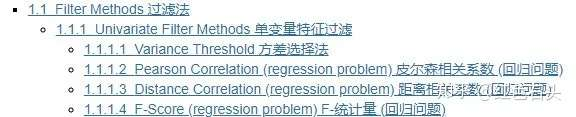## 1.1 Filter Methods 过滤法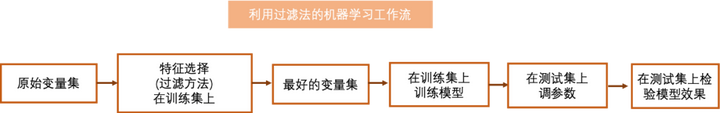### 1.1.1 Univariate Filter Methods 单变量特征过滤

#### 1.1.1.1 Variance Threshold 方差选择法

import numpy as np
import pandas as pd
from sklearn.feature_selection import VarianceThreshold

# 合成一些数据集用于演示
train_set = np.array([[1,2,3],[1,4,7],[1,4,9]]) # 可见第一个变量方差为0
# array([[1, 2, 3],
#        [1, 4, 7],
#        [1, 4, 9]])

test_set = np.array([[3,2,3],[1,2,7]]) # 故意将第二个变量方差设为0
# array([[3, 2, 3],
#        [1, 2, 7]])

selector = VarianceThreshold()
selector.fit(train_set) # 在训练集上训练
transformed_train = selector.transform(train_set) # 转换训练集
# 下面为返回结果，可见第一个变量已被删除
# array([[2, 3],
#        [4, 7],
#        [4, 9]])

transformed_test = selector.transform(test_set) # 转换测试集
# 下面为返回结果，可见第一个变量已被删除
# array([[2, 3],
#        [2, 7]])
# 虽然测试集中第二个变量的方差也为0
# 但是我们的选择是基于训练集，所以我们依然删除第一个变量


#### 1.1.1.2 Pearson Correlation (regression problem) 皮尔森相关系数 (回归问题)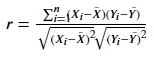import numpy as np
from scipy.stats import pearsonr
from sklearn.feature_selection import SelectKBest

# 直接载入数据集
from sklearn.datasets import fetch_california_housing
dataset = fetch_california_housing()
X, y = dataset.data, dataset.target # 利用 california_housing 数据集来演示
# 此数据集中，X，y均为连续变量，故此满足使用皮尔森相关系数的条件

# 选择前15000个观测点作为训练集
# 剩下的作为测试集
train_set = X[0:15000,:]
test_set = X[15000:,]
train_y = y[0:15000]

# sklearn 中没有直接的方程可以使用
# 此处将用 scipy.stats.pearsonr方程来实现基于皮尔森相关系数的特征过滤
# 注意 scipy.stats.pearsonr 计算的是两个变量之间的相关系数
# 因sklearn SelectKBest需要，我们将基于scipy.stats.pearsonr 重写允许多特征同时输入的方程 udf_pearsonr

def udf_pearsonr(X, y):
# 将会分别计算每一个变量与目标变量的关系
result = np.array([pearsonr(x, y) for x in X.T]) # 包含(皮尔森相关系数, p值) 的列表
return np.absolute(result[:,0]), result[:,1]

# SelectKBest 将会基于一个判别方程自动选择得分高的变量
# 这里的判别方程为皮尔森相关系数
selector = SelectKBest(udf_pearsonr, k=2) # k => 我们想要选择的变量数
selector.fit(train_set, train_y) # 在训练集上训练
transformed_train = selector.transform(train_set) # 转换训练集
transformed_train.shape #(15000, 2), 其选择了第一个及第七个变量
assert np.array_equal(transformed_train, train_set[:,[0,6]])

transformed_test = selector.transform(test_set) # 转换测试集
assert np.array_equal(transformed_test, test_set[:,[0,6]]);
# 可见对于测试集，其依然选择了第一个及第七个变量

# 验算一下我们的结果
for idx in range(train_set.shape):
pea_score, p_value = pearsonr(train_set[:,idx], train_y)
print(f"第{idx + 1}个变量和目标的皮尔森相关系数的绝对值为{round(np.abs(pea_score),2)}, p-值为{round(p_value,3)}")
# 应选择第一个及第七个变量


#### 1.1.1.3 Distance Correlation (regression problem) 距离相关系数 (回归问题)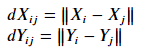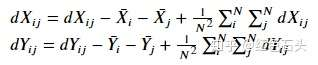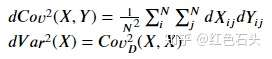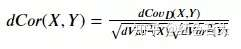import numpy as np
from dcor import distance_correlation
from dcor.independence import distance_covariance_test
from sklearn.feature_selection import SelectKBest

# 直接载入数据集
from sklearn.datasets import fetch_california_housing
dataset = fetch_california_housing()
X, y = dataset.data, dataset.target # 利用 california_housing 数据集来演示
# 此数据集中，X，y均为连续变量，故此满足使用距离相关系数的条件

# 选择前15000个观测点作为训练集
# 剩下的作为测试集
train_set = X[0:15000,:]
test_set = X[15000:,]
train_y = y[0:15000]

# sklearn 中没有直接的方程可以使用
# 此处将用 dcor.distance_correlation方程来实现基于距离相关系数的特征过滤
# 注意 dcor.distance_correlation 计算的是两个变量之间的相关系数
# 因sklearn SelectKBest需要，我们将基于dcor.distance_correlation 重写允许多特征同时输入的方程 udf_dcorr

def udf_dcorr(X, y):
# 将会分别计算每一个变量与目标变量的关系
result = np.array([[distance_correlation(x, y),
distance_covariance_test(x,y)]for x in X.T]) # 包含(距离相关系数, p值) 的列表
return result[:,0], result[:,1]

# SelectKBest 将会基于一个判别方程自动选择得分高的变量
# 这里的判别方程为距离相关系数
selector = SelectKBest(udf_dcorr, k=2) # k => 我们想要选择的变量数
selector.fit(train_set, train_y) # 在训练集上训练
transformed_train = selector.transform(train_set) # 转换训练集
transformed_train.shape #(15000, 2), 其选择了第一个及第三个变量
assert np.array_equal(transformed_train, train_set[:,[0,2]])

transformed_test = selector.transform(test_set) # 转换测试集
assert np.array_equal(transformed_test, test_set[:,[0,2]]);
# 可见对于测试集，其依然选择了第一个及第三个变量

# 验算一下我们的结果
for idx in range(train_set.shape):
d_score = distance_correlation(train_set[:,idx], train_y)
p_value = distance_covariance_test(train_set[:,idx], train_y)
print(f"第{idx + 1}个变量和目标的距离相关系数为{round(d_score,2)}, p-值为{round(p_value,3)}")
# 应选择第一个及第三个变量


#### 1.1.1.4 F-Score (regression problem) F-统计量 (回归问题)

F统计量（F-Score）用于检验线性回归模型的整体显著性。在sklearn中，其将对每一个变量分别建立一个一元的线性回归模型，然后分别报告每一个对应模型的F统计量。F-统计量的零假设是该线性模型系数不显著，在一元模型中，该统计量能够反映各变量与目标变量之间的线性关系。因此，我们应该选择具有较高F统计量的特征（更有可能拒绝原假设）。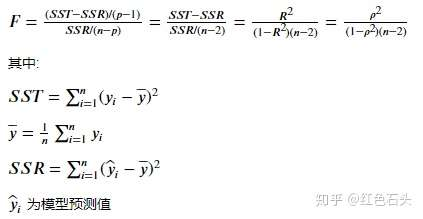SST为总平方和，SSR为回归平方和，p为线性回归自变量数（包括常数项，故在上述的一元线性模型中，p=2）， 为自变量与因变量的线性相关系数，n为总观测数。因上述线性模型为一元线性模型，故可证 2= 2 。

import numpy as np
from sklearn.feature_selection import f_regression
from sklearn.feature_selection import SelectKBest

# 直接载入数据集
from sklearn.datasets import fetch_california_housing
dataset = fetch_california_housing()
X, y = dataset.data, dataset.target # 利用 california_housing 数据集来演示
# 此数据集中，X，y均为连续变量，故此满足使用F统计量的条件

# 选择前15000个观测点作为训练集
# 剩下的作为测试集
train_set = X[0:15000,:]
test_set = X[15000:,]
train_y = y[0:15000]

# sklearn 中直接提供了方程用于计算F统计量
# SelectKBest 将会基于一个判别方程自动选择得分高的变量
# 这里的判别方程为F统计量
selector = SelectKBest(f_regression, k=2) # k => 我们想要选择的变量数
selector.fit(train_set, train_y) # 在训练集上训练
transformed_train = selector.transform(train_set) # 转换训练集
transformed_train.shape #(15000, 2), 其选择了第一个及第七个变量
assert np.array_equal(transformed_train, train_set[:,[0,6]])

transformed_test = selector.transform(test_set) # 转换测试集
assert np.array_equal(transformed_test, test_set[:,[0,6]]);
# 可见对于测试集，其依然选择了第一个及第七个变量

# 验算一下我们的结果
for idx in range(train_set.shape):
score, p_value = f_regression(train_set[:,idx].reshape(-1,1), train_y)
print(f"第{idx + 1}个变量的F统计量为{round(score,2)}, p-值为{round(p_value,3)}")
# 故应选择第一个及第七个变量### 觉得文章有用就打赏一下文章作者

#### 支付宝扫一扫打赏#### 微信扫一扫打赏